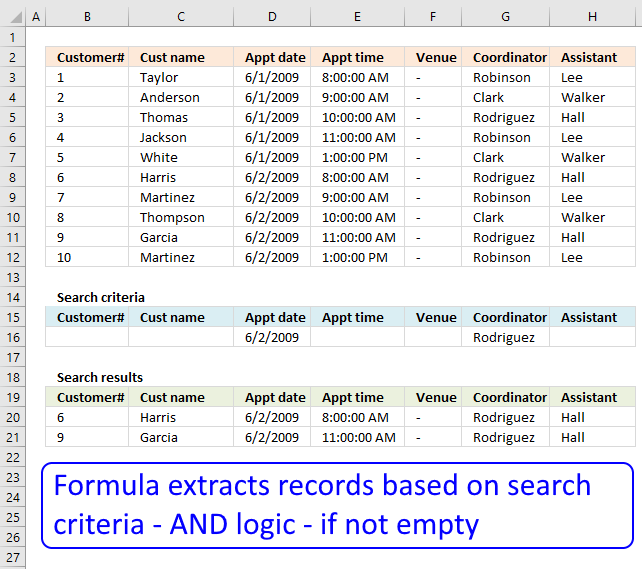Author: Oscar Cronquist Article last updated on December 05, 2018

Question: I second G's question: can this be done for more than 3?

i.e.
(Instead of last name, middle, first)
customer#, cust name, appt date, appt time, venue, coordinator, assistant

A question asked in this post:
Lookup with multiple criteria and display multiple search results using excel formula, part 3Array formula in B20:

=INDEX(\$B\$3:\$H\$12, SMALL(IF(MMULT((\$B\$3:\$H\$12=\$B\$16:\$H\$16)*1, {1;1;1;1;1;1;1})=COUNTA(\$B\$16:\$H\$16), MATCH(ROW(\$B\$3:\$B\$12), ROW(\$B\$3:\$B\$12)), ""), ROWS(\$A\$1:A1)), COLUMNS(\$A\$1:A1))

To enter an array formula, type the formula in a cell then press and hold CTRL + SHIFT simultaneously, now press Enter once. Release all keys.

The formula bar now shows the formula with a beginning and ending curly bracket telling you that you entered the formula successfully. Don't enter the curly brackets yourself.

### Explaining formula in cell B20

#### Step 1 - Compare criteria to data

The equal sign allows you to compare values, the resulting array contains TRUE or FALSE.

(\$B\$3:\$H\$12=\$B\$16:\$H\$16)*1

becomes

({1, "Taylor", 39965, 0.333333333333333, "-", "Robinson", "Lee";2, "Anderson", 39965, 0.375, "-", "Clark", "Walker";3, "Thomas", 39965, 0.416666666666667, "-", "Rodriguez", "Hall";4, "Jackson", 39965, 0.458333333333333, "-", "Robinson", "Lee";5, "White", 39965, 0.541666666666667, "-", "Clark", "Walker";6, "Harris", 39966, 0.333333333333333, "-", "Rodriguez", "Hall";7, "Martinez", 39966, 0.375, "-", "Robinson", "Lee";8, "Thompson", 39966, 0.416666666666667, "-", "Clark", "Walker";9, "Garcia", 39966, 0.458333333333333, "-", "Rodriguez", "Hall";10, "Martinez", 39966, 0.541666666666667, "-", "Robinson", "Lee"}={0, 0, 39966, 0, 0, "Rodriguez", 0})*1

becomes

{FALSE, FALSE, FALSE, FALSE, FALSE, FALSE, FALSE;FALSE, FALSE, FALSE, FALSE, FALSE, FALSE, FALSE;FALSE, FALSE, FALSE, FALSE, FALSE, TRUE, FALSE;FALSE, FALSE, FALSE, FALSE, FALSE, FALSE, FALSE;FALSE, FALSE, FALSE, FALSE, FALSE, FALSE, FALSE;FALSE, FALSE, TRUE, FALSE, FALSE, TRUE, FALSE;FALSE, FALSE, TRUE, FALSE, FALSE, FALSE, FALSE;FALSE, FALSE, TRUE, FALSE, FALSE, FALSE, FALSE;FALSE, FALSE, TRUE, FALSE, FALSE, TRUE, FALSE;FALSE, FALSE, TRUE, FALSE, FALSE, FALSE, FALSE}*1

The MMULT function can't work with boolean values so in order to get that working we must multiply the array with 1.

{0, 0, 0, 0, 0, 0, 0;0, 0, 0, 0, 0, 0, 0;0, 0, 0, 0, 0, 1, 0;0, 0, 0, 0, 0, 0, 0;0, 0, 0, 0, 0, 0, 0;0, 0, 1, 0, 0, 1, 0;0, 0, 1, 0, 0, 0, 0;0, 0, 1, 0, 0, 0, 0;0, 0, 1, 0, 0, 1, 0;0, 0, 1, 0, 0, 0, 0}

#### Step 2 - Add values row-wise

MMULT((\$B\$3:\$H\$12=\$B\$16:\$H\$16)*1,{1;1;1;1;1;1;1})

becomes

MMULT({0, 0, 0, 0, 0, 0, 0;0, 0, 0, 0, 0, 0, 0;0, 0, 0, 0, 0, 1, 0;0, 0, 0, 0, 0, 0, 0;0, 0, 0, 0, 0, 0, 0;0, 0, 1, 0, 0, 1, 0;0, 0, 1, 0, 0, 0, 0;0, 0, 1, 0, 0, 0, 0;0, 0, 1, 0, 0, 1, 0;0, 0, 1, 0, 0, 0, 0},{1;1;1;1;1;1;1})

and returns

{0;0;1;0;0;2;1;1;2;1}

#### Step 3 - Compare sum with the number of criteria

We know a record match if the number of criteria equals the sum returned from the MMULT function. The COUNTA function lets you count non empty cells in a given cell range.

MMULT((\$B\$3:\$H\$12=\$B\$16:\$H\$16)*1, {1;1;1;1;1;1;1})=COUNTA(\$B\$16:\$H\$16)

becomes

{0;0;1;0;0;2;1;1;2;1}=COUNTA(\$B\$16:\$H\$16)

becomes

{0;0;1;0;0;2;1;1;2;1}=2

and returns

{FALSE; FALSE; FALSE; FALSE; FALSE; TRUE; FALSE; FALSE; TRUE; FALSE}.

#### Step 4 - Replace TRUE with corresponding row number

The IF function allows you to return a value if the logical expression evaluates to TRUE and another if FALSE.

IF(MMULT((\$B\$3:\$H\$12=\$B\$16:\$H\$16)*1, {1;1;1;1;1;1;1})=COUNTA(\$B\$16:\$H\$16), MATCH(ROW(\$B\$3:\$B\$12), ROW(\$B\$3:\$B\$12)), "")

becomes

IF({FALSE; FALSE; FALSE; FALSE; FALSE; TRUE; FALSE; FALSE; TRUE; FALSE}, MATCH(ROW(\$B\$3:\$B\$12), ROW(\$B\$3:\$B\$12)), "")

becomes

IF({FALSE; FALSE; FALSE; FALSE; FALSE; TRUE; FALSE; FALSE; TRUE; FALSE}, {1;2;3;4;5;6;7;8;9;10}, "")

and returns

{"";"";"";"";"";6;"";"";9;""}

#### Step 5 - Extract the k-th smallest row number

The SMALL function lets you get the k-th smallest number in an array. SMALL( array, k)

SMALL(IF(MMULT((\$B\$3:\$H\$12=\$B\$16:\$H\$16)*1, {1;1;1;1;1;1;1})=COUNTA(\$B\$16:\$H\$16), MATCH(ROW(\$B\$3:\$B\$12), ROW(\$B\$3:\$B\$12)), ""), ROWS(\$A\$1:A1))

becomes

SMALL({"";"";"";"";"";6;"";"";9;""}, ROWS(\$A\$1:A1))

The ROWS function returns the number of rows in a cell reference, this particular cell reference is expanding when the cell is copied to cells below.

SMALL({"";"";"";"";"";6;"";"";9;""}, 1)

and returns 6.

#### Step 6 - Return value

The INDEX function returns a value from a cell range or array based on a row and column number.

INDEX(\$B\$3:\$H\$12, SMALL(IF(MMULT((\$B\$3:\$H\$12=\$B\$16:\$H\$16)*1, {1;1;1;1;1;1;1})=COUNTA(\$B\$16:\$H\$16), MATCH(ROW(\$B\$3:\$B\$12), ROW(\$B\$3:\$B\$12)), ""), ROWS(\$A\$1:A1)), COLUMNS(\$A\$1:A1))

becomes

INDEX(\$B\$3:\$H\$12, 6, COLUMNS(\$A\$1:A1))

becomes

INDEX(\$B\$3:\$H\$12, 6, 1)

and returns "6" in cell B20.

### Get Excel *.xlsx file

Lookup-with-multiple-criteria-and-display-multiple-search-results-using-excel-formula-part-4-johnv2.xlsx